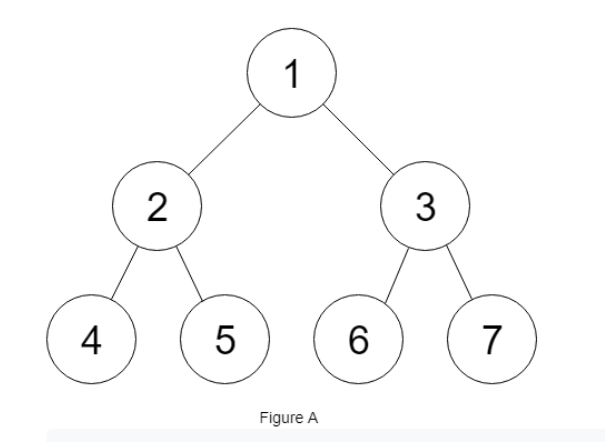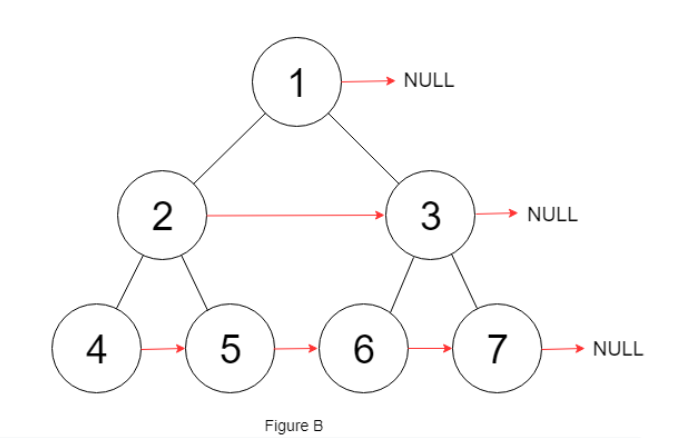×

Search anything:

# Connect Nodes at Same Level in Binary Tree

#### Problems on Binary Tree AlgorithmsGet this book -> Problems on Array: For Interviews and Competitive Programming

In this article, we will be discussing about the problem "Connect Nodes at Same Level in a Binary Tree" in detail. This involve the concept of Breadth First Search (BFS) or Level Order Traversal.

Contents:

1. Introduction
2. Optimized approach
3. Implementation
4. Code Explanation
5. Time and Space Complexity Analysis

## Introduction:

In this problem at OpenGenus, we are going to connect the nodes at same level in a binary tree. we'll be given an addition nextRight pointer for the same and we have to connect all the adjacent nodes at the same level starting from the left-most node of that level, and ending at the right-most node using this nextRight pointer.
Before connecting all the nextPointer of each node to its next right it points to a garbage value. So, we have to create a function that should point this nextRight pointers to next right node for each node.

Example:Suppose we are given a binary tree, and our task is to connect each nextRight pointer to its next right node at a same level. If a particular node is at the end of a particular level then we have to point the nextRight pointer to null.The above figure shows how we have to connect nextRight pointer of each node.

## Optimized Approach:

In the optimized approach, we will traverse the given binary tree in a breadth first search manner or level order traversal using queue data structure. For each level of traversal we will connect each node of a particular level to its next right node. The nextRight pointer of each node will point to the next adjacent node in the right of it. After traversing the whole tree we will return the updated binary tree.

Algorithm:

1. Traverse the given binary tree in a level order manner using queue data structure.
2. Create a variable count which will store the total number of variables in a particular level.
count = q.size();
3. Connect each node of a particular level with its next right node. The next of a particular node will be the next element in the queue.
nextRight = q.peek();
4. If a particular node is the last element of a particular level, then make nextRight pointer of that node point to null.
if(i==count-1){
nextRight = null;
}

## Implementation:

``````import java.util.*;
class Node{
int data;
Node left;
Node right;
Node nextRight;
Node(int data){
this.data = data;
left=null;
right=null;
nextRight = null;
}
}

class Solution
{
//Function to connect nodes at same level.
public static void connect(Node root)
{
if(root!=null){
}
while(q.isEmpty()==false){
int count = q.size();
for(int i = 0; i<count;i++){
Node curr = q.poll();
if(curr.left!=null){
}
if(curr.right!=null){
}
if(i==count-1){
curr.nextRight = null;
}
else{
curr.nextRight = q.peek();
}

}

}

}
public static void main(String args[])
{
BinaryTree tree = new BinaryTree();

/* Constructed binary tree is
10
/  \
8     2
/
3
*/
Node root = new Node(10);
root.left = new Node(8);
root.right = new Node(2);
root.left.left = new Node(3);

connect(root);

System.out.println(
"Following are populated nextRight pointers in "
+ "the tree"
+ "(-1 is printed if there is no nextRight)");
int a = root.nextRight != null
? root.nextRight.data
: -1;
System.out.println("nextRight of " + root.data
+ " is " + a);
int b = root.left.nextRight != null
?root.left.nextRight.data
: -1;
System.out.println("nextRight of "
+ root.left.data + " is "
+ b);
int c = root.right.nextRight != null
? root.right.nextRight.data
: -1;
System.out.println("nextRight of "
+ root.right.data + " is "
+ c);
int d = root.left.left.nextRight != null
? root.left.left.nextRight.data
: -1;
System.out.println("nextRight of "
+ root.left.left.data
+ " is " + d);
}
}

``````

## Code Explanation:

1. We are creating a function connect() and pass the root node as a parameter in this.
2. This function will return the updated binary tree in which every node is connected by its next right node by nextRight pointer by using level order traversal.
3. If you don't know how level order traversal of a binary tree works using a queue data structure, first you can go through this https://iq.opengenus.org/level-order-traversal-binary-tree/.
4. In level order traversal, if we are traversing the last node of a particular level then all the node of its next level must have been added to the queue till then and all the nodes of that level must been removed from the queue.
5. So we will be creating a variable count to get the total count of nodes at a particular level and will run the loop count times.
6. If the value of i is equals to the value of count, it means that it is the last node of a particular level. so we the point nextRight of that node to null.
7. Otherwise we will point nextRight of every node to next element in the queue by using q.peek() function, where q is the queue of type node.
8. If the queue becomes empty, then we have traversed the whole binary tree. Therefore, we will return from the function call.

## Time and Space Complexity Analysis:

The time complexity of the above solution will be O(N),where N is total number of nodes in the binary tree. As in the above approach we are doing complete traversal of the binary tree, so the overall complexity of the above solution will be O(N).

The space complexity of the above solution will be O(N), where N is total number of nodes in the binary tree. Since we are creating a queue data structure and at a time the maximum nodes that the queue will store is the all nodes of a particular level which will be maximum width of the binary tree. The maximum width of binary tree can be N/2 if it is complete binary tree, so the overall space complexity of the above solution will be O(N).

With this article at OpenGenus, you must have the complete idea of solving this problem "Connect Nodes at Same Level in a Binary Tree".

#### Uddeshya Raj

Uddeshya Raj is pursuing B. Tech in Computer Science from Chandigarh University. He is an Algorithm and Data Structure Developer, Intern at OpenGenus.